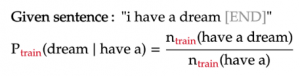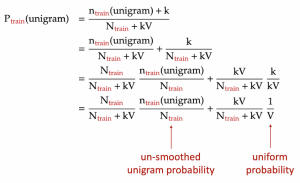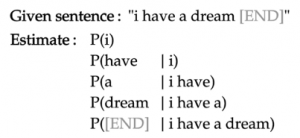# Background

In part 1 of my project, I built a unigram language model: it estimates the probability of each word in a text simply based on the fraction of times the word appears in that text.

The text used to train the unigram model is the book “A Game of Thrones” by George R. R. Martin (called train). The texts on which the model is evaluated are “A Clash of Kings” by the same author (called dev1), and “Gone with the Wind” — a book from a completely different author, genre, and time (called dev2).

In this part of the project, I will build higher n-gram models, from bigram (n=2) to 5-gram (n=5). These models are different from the unigram model in part 1, as the context of earlier words is taken into account when estimating the probability of a word.

# Higher n-gram language models

## Training the model

For a given n-gram model:

The example below shows how to calculate the probability of a word in a trigram model:For simplicity, all words are lower-cased in the language model, and punctuations are ignored. The presence of the [END] tokens is explained in part 1.

# Dealing with words near the start of a sentence

In higher n-gram language models, the word near the start of each sentence will not have a long enough context to apply the formula above. To make the formula consistent for those cases, we will pad these n-grams with sentence-starting symbols [S]. Below are two such examples under the trigram model:

From the above formulas, we see that the n-grams containing the starting symbols are just like any other n-gram. The only difference is that we count them only when they are at the start of a sentence. Lastly, the count of n-grams containing only [S] symbols is naturally the number of sentences in our training text:

# Dealing with unknown n-grams

Similar to the unigram model, the higher n-gram models will encounter n-grams in the evaluation text that never appeared in the training text. This can be solved by adding pseudo-counts to the n-grams in the numerator and/or denominator of the probability formula a.k.a. Laplace smoothing. However, as outlined in part 1 of the project, Laplace smoothing is nothing but interpolating the n-gram model with a uniform model, the latter model assigns all n-grams the same probability:Laplace smoothing for unigram model: each unigram is added a pseudo-count of k. N: total number of words in the training text. V: number of unique unigrams in the training text.

Hence, for simplicity, for an n-gram that appears in the evaluation text but not the training text, we just assign zero probability to that n-gram. Later, we will smooth it with the uniform probability.

# Background

Language modeling — that is, predicting the probability of a word in a sentence — is a fundamental task in natural language processing. It is used in many NLP applications such as autocompletespelling correction, or text generation.

Currently, language models based on neural networks, especially transformers, are the state of the art: they predict very accurately a word in a sentence based on surrounding words. However, in this project, I will revisit the most classic language model: the n-gram models.

# Data

In this project, my training data set — appropriately called train — is “A Game of Thrones”, the first book in the George R. R. Martin fantasy series that inspired the popular TV show of the same name.

Then, I will use two evaluating texts for our language model:

# Unigram language model

## What is a unigram?

In natural language processing, an n-gram is a sequence of n words. For example, “statistics” is a unigram (n = 1), “machine learning” is a bigram (n = 2), “natural language processing” is a trigram (n = 3), and so on. For longer n-grams, people just use their lengths to identify them, such as 4-gram, 5-gram, and so on. In this part of the project, we will focus only on language models based on unigrams i.e. single words.

## Training the model

A language model estimates the probability of a word in a sentence, typically based on the words that have come before it. For example, for the sentence “I have a dream”, our goal is to estimate the probability of each word in the sentence based on the previous words in the same sentence:For simplicity, all words are lower-cased in the language model, and punctuations are ignored. The [END] token marks the end of the sentence and will be explained shortly.

The unigram language model makes the following assumptions:

# Background

In previous parts of my project, I built different n-gram models to predict the probability of each word in a given text. This probability is estimated using an n-gram — a sequence of words of length n — which contains the word. The below formula shows how the probability of the word “dream” is estimated as part of the trigram “have a dream”:

We train the n-gram models on the book “A Game of Thrones” by George R. R. Martin (called ). We then evaluate the models on two texts: “A Clash of Kings” by the same author (called ), and “Gone with the Wind” — a book from a completely different author, genre, and time (called ).

The metric to evaluate the language model is average log-likelihood: the average of the log probability that the model assigns to each word in the evaluation text.

Often, the log of base 2 is applied to each probability, as is the case in the first two parts of the project. Nevertheless, in this part, I will use a natural log, as it makes it simpler to derive the formulas that we will be using.

# Problem

In part 2, the various n-gram models — from unigram to 5-gram — were evaluated on the evaluation texts ( and , see graphs below).

From this, we notice that:

• The Bigram model performs slightly better than the unigram model. This is because the previous word to the bigram can provide important context to predict the probability of the next word.
• Surprisingly, the trigram model and up is much worse than bigram or unigram models. This is largely due to the high number of trigrams, 4-grams, and 5-grams that appear in the evaluation texts but nowhere in the training text. Hence, their predicted probability is zero.
• For most n-gram models, their performance is slightly improved when we interpolate their predicted probabilities with the uniform model. This seems rather counter-intuitive since the uniform model simply assigns equal probability to every word. However, as explained in part 1, interpolating with this “dumb” model reduces the overfit and variance of the n-gram models, helping them generalize better to the evaluation texts.

In this part of the project, we can extend model interpolation even further: instead of separately combining each n-gram model with the uniform, we can interpolate different n-gram models with one another, along with the uniform model.

# What to interpolate?

The first question to ask when interpolating multiple models together is:

To answer this question, we use the simple strategy outlined below:

1. First, we start with the uniform model. This model will have very low average log-likelihoods on the evaluation texts since it assigns every word in the text the same probability.
2. Next, we interpolate this uniform model with the unigram model and re-evaluate it on the evaluation texts. We naively assume that the models will have equal contribution to the interpolated model. As a result, each model will have the same interpolation weight of 1/2.
3. We then add the bigram model to the mix. Similarly, in this 3-model interpolation, each model will simply have the same interpolation weight of 1/3.
4. We keep adding higher n-gram models to the mix while keeping the mixture weights the same across model, until we reach the 5-gram model. After each addition, the combined model will be evaluated against the two evaluation texts,  and .

# Coding the interpolation

In part 2, each evaluation text had a corresponding probability matrix. This matrix has 6 columns — one for each model — and each row of the matrix represents the probability estimates of each word under the 6 models. However, since we want to optimize the model performance on both evaluation texts, we will vertically concatenate these probability matrices into one big evaluation probability matrix (803176 rows × 6 columns):